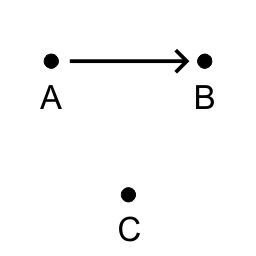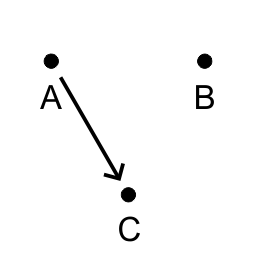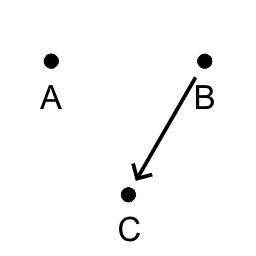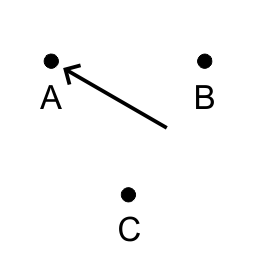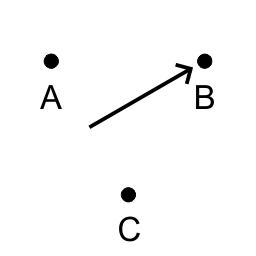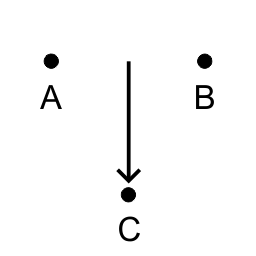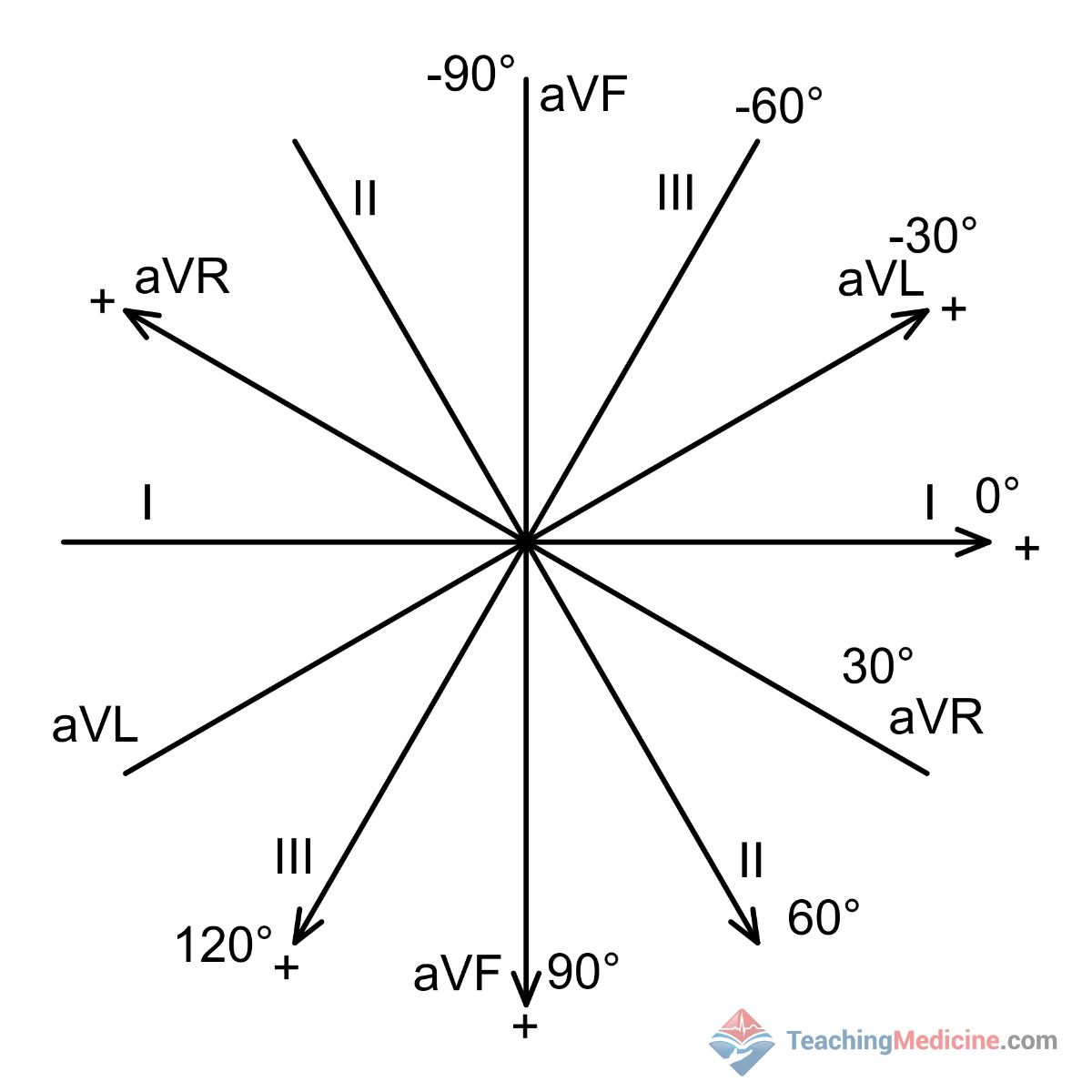ECGLevel 3Tutorial: AxisTutorial: Axis
This will teach a basic approach to determining the axis of an ECG.
How to level up?
Develop your skills by completing our Practice Cases!
Lessons42
Times Practiced1284
Cases Completed1h 24m
Total Time spent1m 24s
Average Time
Progress
Accuracy
Efficiency
AccuracyEfficiency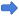1 Remember the Vector 2 Quadrants 3 Defining the Normal axis 4 The Isoelectric lead 5 Shading the QRS
Remember the Vector
This module assumes you have completed all modules on introduction to ECG and have successfully completed the ECG Rhythm Practice modules.

This module will teach about the electrical axis. This topic causes confusion for many learners, so if you find some parts confusing, please click on the Feedback  button (top right of screen) to let us know how to improve the lessons.

In the module "Tutorial: Basic Electric Stuff", we covered the topic of vectors. That lesson is a pre-requisite for this module.

Remember that a vector represents the size and direction of movement of an electric charge. The QRS vector is sum of all the little cellular charges that occur during ventricular depolarization. There are vectors for the P wave and T wave as well but we will not discuss those here.
If you understand the concept of vectors, then the concept of axis is not very different.

Axis is the "average" vector direction. If the electrical activity is MOSTLY moving in one direction, then that direction will be the axis.

So how do we determine the direction of the axis? The answer is by analyzing the QRS complex in different ECG leads. Note that we have 12 leads and the 6 limb leads measure in a right-left and head-toe direction and therefore defines a plane, called the coronal plane:Remember that these are the 6 limb leads: